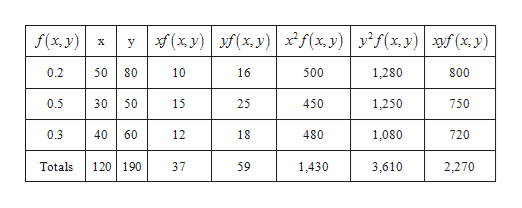5) Given below is a bivariate distribution for the random variables x and y.f(x, y)xy0.250800.530500.34060Compute the covariance and correlation for x and y. Are x and y positively related, negatively related, or unrelated?Is the variance of the sum of x and y bigger, smaller, or the same as the sum of the individual variances? Why?

Question

5) Given below is a bivariate distribution for the random variables x and y.

 f(x, y) x y 0.2 50 80 0.5 30 50 0.3 40 60
1. Compute the covariance and correlation for x and y. Are x and y positively related, negatively related, or unrelated?
2. Is the variance of the sum of x and y bigger, smaller, or the same as the sum of the individual variances? Why?
Step 1

Obtain the mean of x and mean of y:

The mean of x is, sum(xf(x.y) =E(x)=37 and mean of y is, sum(yf(x.y)=E(y) =59help_outlineImage Transcriptionclose(xy) f(x y)f(x, y) yxy)f (x y) f(x, y) X y 0.2 800 80 10 16 500 1,280 1,250 0.5 30 50 15 25 450 750 0.3 40 60 12 18 480 1,080 720 120 190 Totals 37 59 1,430 3,610 2,270 fullscreen
Step 2

Obtain the variance of x:

The variance of x is,

Step 3

Obtain the variance of y:

The varian...

Want to see the full answer?

See Solution

Want to see this answer and more?

Our solutions are written by experts, many with advanced degrees, and available 24/7

See Solution
Tagged in

Other